# Microwave Absorbing Features of Ce2(Co0.3Fe0.7)17/Ferrite Coating Material

Microwave Absorbing Features of Ce2(Co0.3Fe0.7)17/Ferrite Coating Material

Beijing Special Engineering Design and Research Institute, Beijing 100028, China

Corresponding Author Email:
wjq808@163.com
Page:
39-44
|
DOI:
https://doi.org/10.18280/rcma.290107
23 October 2018
|
Accepted:
5 January 2019
|
Published:
30 April 2019
| Citation

OPEN ACCESS

Abstract:

This paper attempts to combine Ce2(Co0.3Fe0.7)17 and Co2Z-type hexagonal ferrite (hereinafter referred to the ferrite) into a composite with excellent absorbing properties. Firstly, the permittivity and permeability spectra of Ce2(Co0.3Fe0.7)17/epoxy resin composite were investigated, so were those of the ferrite/epoxy resin composite. On this basis, the author prepared coaxial Ce2(Co0.3Fe0.7)17/ferrite/epoxy resin composite specimens at different volume ratios of the Ce2(Co0.3Fe0.7)17 particles and the ferrite particles, and also studied their permittivity and permeability spectra in the frequency range of 8~18 GHz. In this way, the optimal volume ratios of both types of particles were determined for the composite. Through the analysis, it is concluded that, based on the matrix of epoxy resin and polyamide, the single-layer plate absorber specimens can control the reflection loss within -10dB across the frequency range 8~18GHz, when the thickness is 1.1 mm, the fraction volume of Ce2(Co0.3Fe0.7)17 is 25 % and the fraction volume of ferrite is 20 %.

Keywords:

absorbent, ferrite, reflection loss, coating material, composite

1. Introduction

With the proliferation of modern communication technology, electromagnetic devices have penetrated every corner of our life and propagated to both civil and military fields. This calls for full protection of these devices to eliminate electromagnetic interference and ensure electromagnetic compatibility. One of the most popular methods for electromagnetic protection lies in the adoption of absorbents [1-8]. In military field, the absorbent must be sufficiently thin, light, wide and strong to shield electromagnetic interference.

According to the theories on transmission line and quarter wavelength [9-12], the properties of absorbents (e.g. thickness) are heavily influenced by permittivity and permeability. The rare-earth transition-metal intermetallic compound Ce2(Co0.3Fe0.7)17  offers a viable solution to reduce the dosage and thickness of absorbents, thanks to excellent performance in a wide range and at high frequencies, which can break through the Snoek limitation. However, the high permittivity of this material hinders the impedance matching with the free space, and thus limits its application as absorbent. Considering the small permittivity of the Co2Z-type hexagonal ferrite, this paper attempts to combine Ce2(Co0.3Fe0.7)17 and Co2Z-type hexagonal ferrite into a composite with excellent absorbing properties.

2. Methodology

2.1 Materials

The absorbent was prepared from Ce2(Co0.3Fe0.7)17 (particle diameter: 10~50μm) with in-plane anisotropy and Co2Z-type hexagonal ferrite (hereinafter referred to as ferrite). The coating material is square aluminum plate (length: 18mm). The matrix resin includes epoxy resin (E44) and polyamide (650).

2.2 Coaxial specimens

First, epoxy resin and polyamide were weighed at the mass ratio of 1:1, mixed into the matrix resin at different volume fractions, and relocated into a crucible. Then, the n-butyl alcohol was added into the matrix resin to dissolve the epoxy resin and polyamide. After that, the mixture was dispersed for 5min with an ultrasonic cleaner (KQ218). Next, the prepared absorbent was relocated into a crucible, and dispersed continuously for 1.5h with an ultrasonic cleaner (KQ218). After ultrasonic dispersion, the absorbent/resin compound was extracted from the crucible and cut into granular particles. The particles were then put into a coaxial mold (inner diameter: 3.04 mm; external diameter: 7.00 mm) and compressed for 8h by tablet compressing machine (FW-4) under 2MPa. Three specimens were thus prepared for each volume fraction.

2.3 Coating specimens

The prepared absorbent was separately added into epoxy resin and polyamide, and dispersed to prepare a coating material of two components. Each component was dispersed with a high-speed grinder (SKL-FS400). For the epoxy resin component, the epoxy resin, absorbent and zirconium beads were added into a tank in turn and stirred at a low speed (<500r/min). Then, the stirring speed was gradually raised to 4,000r/min. Meanwhile, the mixed solvent of xylene and n-butyl alcohol (mass ratio of 5:1) was added into the tank. After a 4h dispersion, the zirconium beads were washed out by the same mixed solvent. The polyamide component went through the same dispersion process. Next, the two dispersed components were sprayed into coating specimens, and left solidified at room temperature.

2.4 Reflection loss test

The reflection loss was tested by Bow Reflectivity Testing Method at the frequency between 8 and 18GHz. The dielectric constant $ε_r$ (${{\varepsilon }_{r}}=(\varepsilon _{r}^{'}-j\varepsilon _{r}^{''})$) and permeability $μ_r$(${{\mu }_{r}}({{\mu }_{r}}=\mu _{r}^{'}-j\mu _{r}^{''})$)of the coaxial specimens were tested through vector network analysis at the frequency between 0.1 and 18GHz.

3. Results and Discussion

3.1 Electromagnetic spectrum features of Ce2(Co0.3Fe0.7)17/resin composite

## 11.jpg## 12.jpg## 13.jpg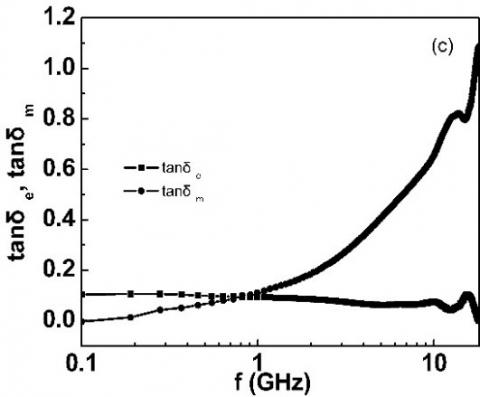Figure 1. Frequency dependence of permittivity, permeability and of Ce2(Co0.3Fe0.7) 17/resin composite

Figure 1 shows the variations in permittivity, permeability and loss tangent ($tan⁡δ$) of Ce2(Co0.3Fe0.7)17/resin composite as the frequency increased from 0.1 to 18GHz. The volume fraction of Ce2(Co0.3Fe0.7)17 particles is 25 % in this composite. The $tan⁡δtan⁡δ$ can be calculated by:

$\tan {{\delta }_{e}}=\frac{{{\varepsilon }''}}{{{\varepsilon }'}}$ (1)

$\tan {{\delta }_{\text{m}}}=\frac{{{\mu }''}}{{{\mu }'}}$ (2)

where, $tan⁡δ_e$  and $tan⁡δ_m$  are the loss tangent values obtained from permittivity and permeability, respectively. The two parameters describe the microwave depletion of the composite.

From Figures 1(b) and 1(c), it is clear that both the imaginary permeability and the maximum $tan⁡δ_m$  surpassed one, while the value of $tan⁡δ_e$  stayed below 0.2 at all frequencies. With the growth in frequency, the value of $tan⁡δ_e$  remained basically stable. Meanwhile, the value of $tan⁡δ_m$ exhibited an obvious increase, indicating that Ce2(Co0.3Fe0.7)17 is a magnetic loss absorbing material. As shown in Figure 1 (a), the real permittivity was below 12, and, similar to the imaginary permittivity, decreased with the rise of frequency. This reveals the good impedance matching of the composite. In addition, the resonance peak appeared in the imaginary permeability in Figure 1 (b), which is attributable to the loss of electromagnetic wave propagating in the composite.

3.2 Electromagnetic spectrum features of ferrite/resin composite

## 21.jpg## 22.jpg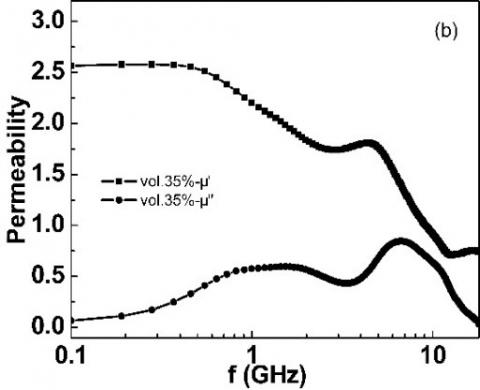## 23.jpgFigure 2. Frequency dependence of permittivity, permeability and $tan⁡δ$ of ferrite/resin composite

Figure 2 presents the variations in permittivity, permeability and $tan⁡δ$ of ferrite/resin composite as the frequency increased from 0.1 to 18 GHz. The volume fraction of ferrite particles is 35 % in this composite. The  tan⁡δ$is also calculated by equations (1) and (2). It can be seen from Figure 2 (a) that the real and imaginary permittivities were respectively smaller than 7.5 and 1. Overall, the permittivity of the ferrite/resin composite is relatively low at a high-volume fraction of ferrite (35 %). As shown in Figure 2(b), the imaginary permeability of ferrite was relatively low but the twin peaks in the curve of imaginary permeability helps to widen the bandwidth of the absorbent. With a low permittivity, this composite can be used to improve the impedance matching of Ce2(Co0.3Fe0.7)17/resin composite by widening the absorption band. 3.3 Microwave absorbing features of ferrite/resin composite Inspired by the theory on transmission line [9-11], the reflection loss of single-layer plate absorber can be derived from the permittivity and permeability with the following equations:$R=20\lg \left| \frac{{{Z}_{in}}(1)-{{Z}_{0}}}{{{Z}_{in}}(1)+{{Z}_{0}}} \right|=20\lg \left| \frac{{{\eta }_{in}}(1)-1}{{{\eta }_{in}}(1)+1} \right|$(3)${{\eta }_{in}}(1)={{\eta }_{1}}tgh(j{{k}_{1}}{{d}_{1}})=\sqrt{\frac{{{\mu }_{r1}}}{{{\varepsilon }_{r1}}}}tgh(j\frac{2\pi f\sqrt{{{\varepsilon }_{r1}}{{\mu }_{r1}}}}{c}{{d}_{1}})$(4) where c is the light velocity; is the frequency of electromagnetic wave; d1 is the thickness of absorbing layer;$ε_r1$and$μ_r1$are the real and imaginary permittivities, respectively. Hence, the microwave absorbing features can be determined based on the relationships between frequency and permittivity and permeability. Figure 3 displays the reflectivity of ferrite/resin single-layer plate absorber with different thicknesses. To fully display the microwave absorbing features of ferrite/resin composite, the frequency dependence of normalized input impedance ($|Z_in/Z_0 |$) and minimum reflectivity when the ferrite/resin composite is of the thicknesses of (1/4)γ and (3/4)γ are displayed in Figure 4, where γ is the wavelength at a certain frequency. As can be seen from Figure 3, the reflection loss was less than-10dB at the frequency of 8.2 GHz, and the minimum reflection loss was -20dB. The results indicate that the ferrite/resin composite enjoys a relatively wide bandwidth. According to Figure 4, the normalized input impedance intersected the line of$|Z_in/Z_0 |=1$(the completely matching line) at two points, revealing that the composite has two completely matching points, respectively at the frequencies of 7.8GHz and 13.9GHz. Between the two points, the normalized input impedance curve was close to one, that is, the composite remains close to the completely matching state under the frequency of 6GHz. This explains the relative wide bandwidth in Figure 3. Due to the small imaginary the permittivity, the absorbing layer must be extremely thick if only ferrite particles are used. The above analysis shows that Ce2(Co0.3Fe0.7)17 particles enjoy good magnetic performance at high frequencies, and cause strong magnetic loss to electromagnetic wave at a small dosage of absorbent or with a thin coating. The ferrite particles can widen the absorption band of the absorbent specimens, thanks to their low permittivity, good impedance matching to free space, and bimodal features of imaginary permeability. If combined, the two types of particles can produce a powerful absorbing coating with broad bandwidth and limited thickness. ## 3.jpgFigure 3. Frequency dependence of reflectivity at different thicknesses ## 4.jpgFigure 4. Frequency dependence of normalized input impedance and minimum reflectivity at the thicknesses of (1/4)γ and (3/4)γ 3.4 Electromagnetic spectrum features of Ce2(Co0.3Fe0.7)17/ferrite/resin composite Figure 5 provides the spectra of permittivity and permeability of coaxial Ce2(Co0.3Fe0.7)17/ferrite/ resin composite at different volume fractions of the two types of particles (the total volume fraction of the two particles is 45 %). It can be seen that the permittivity and permeability, especially the real permittivity, changed greatly with the volume fractions of the two types of particles. The twin peaks in the curve of imaginary permeability come from the ferrite. In general, the permittivity is negatively correlated with the volume fraction of ferrite, while the impedance matching is positively corelated with the latter. ## 51.jpg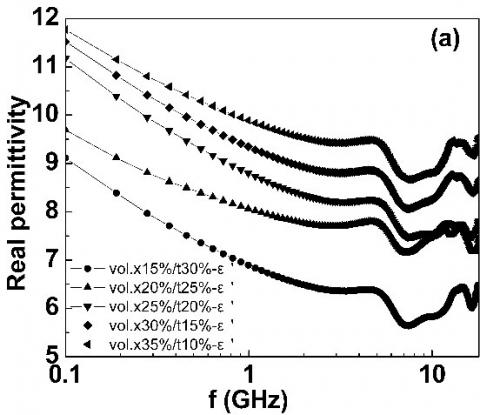## 52.jpg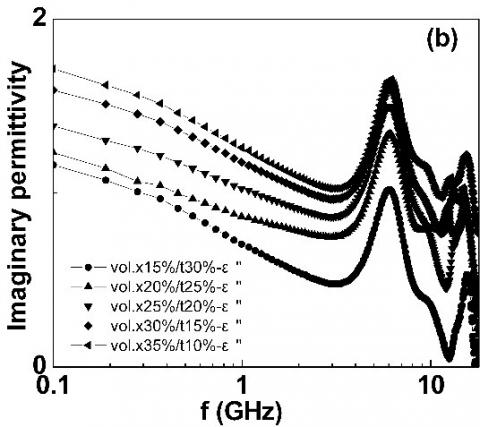## 53.jpg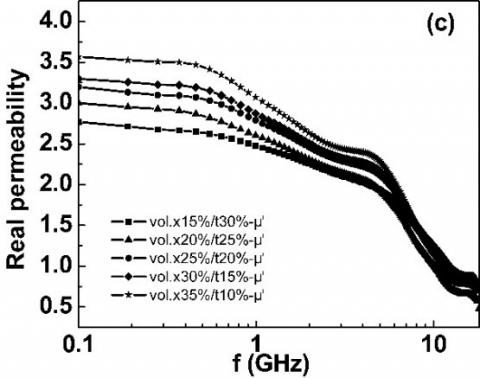## 54.jpg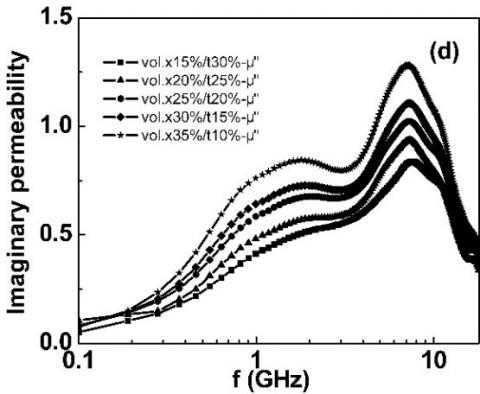Figure 5. The spectra of permittivity and permeability of coaxial Ce2(Co0.3Fe0.7)17/ferrite/resin composite at different volume fractions of Ce2(Co0.3Fe0.7)17 (x) and ferrite (y) 3.5 Microwave absorbing features of Ce2(Co0.3Fe0.7)17/ferrite/resin composite The microwave absorbing features of Ce2(Co0.3Fe0.7)17/ferrite/resin composite were investigated based on the permittivity and permeability spectra in Figure 5. ## 611.jpg## 612.jpg## 613.jpg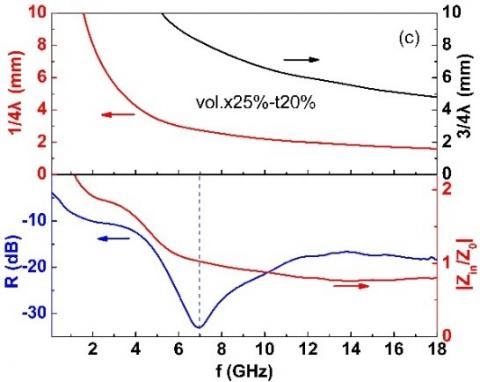## 614.jpg## 615.jpgFigure 6. Frequency dependence of normalized input impedance and minimum reflectivity at the thicknesses of (1/4)γ and (3/4) The results are plotted as Figure 6, where x is Ce2(Co0.3Fe0.7)17 and t is ferrite. It can be seen that the completely matching point shifted towards the low frequency with the growth in the volume fraction of ferrite. Meanwhile, the normalized input impedance curve approached the completely matching line$|Z_in/Z_0 |=1\$ , indicating that the more the ferrite particles, the better the impedance matching and the broader the bandwidth.

3.6 Reflection properties of coating specimens

Based on the volume fractions in Figure 5, the single-layer plate absorber specimens were prepared from 18cm-long square aluminum plates. The reflection losses of these specimens were tested at the frequency between 8 and 18GHz. The test results are given in Figure 7 and Table 1. As shown in Figure 7, the minimum reflectivity of -25dB belonged to specimen 1# (thickness: 1.5mm; Ce2(Co0.3Fe0.7)17 volume fraction: 15 %; ferrite volume fraction: 30 %). The reflection loss of specimen 3# (thickness: 1.1mm; Ce2(Co0.3Fe0.7)17 volume fraction: 25 %; ferrite volume fraction: 20 %) remained less than -10dB across the frequency range, a signal for a broad bandwidth.

## 7.jpg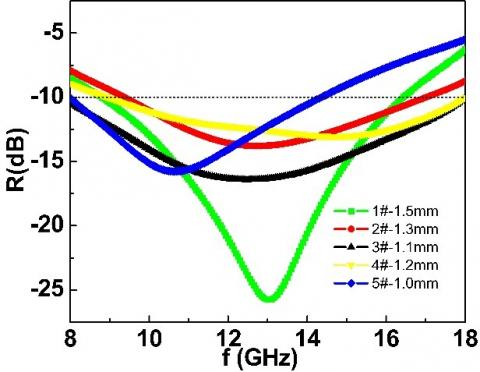Figure 7. Frequency dependence of reflection loss of Ce2(Co0.3Fe0.7)17/ferrite/resin single-layer plate absorber specimens (1#: vol. x15 %/t30 %; 2#: vol. x20 %/t25 %; 3#: vol. x25 %/t20 %; 4#: vol. x30 %/t15%; 5#: vol. x35 %/t10 %)

Table 1. Test results on the specimens

 No. Thickness [mm] Peak reflection [dB] Peak frequency [GHz] Effective bandwidth [GHz] Surface density [kg/m2] 1# 1.5 -25.8 13.1 7.6 3.83 2# 1.3 -13.8 12.8 7.6 3.74 3# 1.1 -16.4 12.5 10.0 3.61 4# 1.2 -13.0 14.7 9.0 3.66 5# 1.0 -15.8 10.7 6.4 3.55

4. Conclusions

(1) The absorbent Ce2(Co0.3Fe0.7)17 boasts good magnetic properties at high frequencies.

(2) The Ce2(Co0.3Fe0.7)17/resin composite has relatively high imaginary permeability, and causes strong magnetic loss. Meanwhile, the real permittivity of the composite is extremely high, and increases quickly with the growth in the volume fraction of Ce2(Co0.3Fe0.7)17.

(3) The ferrite has relatively low permittivity, and moderate and bimodal imaginary permeability. These features are conducive to impedance matching and bandwidth.

(4) Based on the matrix of epoxy resin and polyamide, the single-layer plate absorber specimens can control the reflection loss within -10dB across the frequency range 8~18GHz, when the thickness is 1.1 mm, the fraction volume of Ce2(Co0.3Fe0.7)17 is 25 % and the fraction volume of ferrite is 20 %.

References

 Sadiq I, Naseem S, Ashiq MN, Khan MA, Niaz S, Rana MU. (2016). Tunable microwave absorbing nano-material for X-band applications. Journal of Magnetism and Magnetic Materials 401: 63-69. https://doi.org/10.1016/j.jmmm.2015.09.024

 Seo LS, Chin WS, Lee DG. (2004). Characterization of electromagnetic properties of polymeric composite materials with free space method. Composite Structures 66(1-4): 533-542. https://doi.org/10.1016/j.compstruct.2004.04.076

 Das S, Nayak GC, Sahu SK, Oraon R. (2015). Development of FeCoB/Graphene Oxide based microwave absorbing materials for X-Band region. Journal of Magnetism and Magnetic Materials 384: 224-228. https://doi.org/10.1016/j.jmmm.2015.01.079

 Yusoff AN, Abdullah MH. (2004). Microwave electromagnetic and absorption properties of some LiZn ferrites. Journal of Magnetism and Magnetic Materials 269(2): 271-280. https://doi.org/10.1016/S0304-8853(03)00617-6

 Folgueras LC, Alves MA, Rezende MC. (2014). Evaluation of a nanostructured microwave absorbent coating applied to a glass fiber/polyphenylene sulfide laminated composite. Materials Research 17(1). https://doi.org/10.1590/S1516-14392014005000009

 Panwar R, Puthucheri S, Agarwala V, Singh D. (2015). Fractal frequency-selective surface embedded thin broadband microwave absorber coatings using heterogeneous composites. IEEE Transactions on Microwave Theory and Techniques 63(8): 2438-2448. https://doi.org/10.1109/TMTT.2015.2446989

 Xiong GX, Xu LL, Deng M, Huang HQ, Tang MS. (2005). Research on absorbing EMW properties and mechanical properties of nanometric TiO_2 and cement composites. Journal of Functional Materials & Devices 11(1): 87-91.

 Wei JQ, Zhang ZQ, Wang BC, Wang T, Li F. (2010). Microwave reflection characteristics of surface-modified Fe50Ni50Fe50Ni50 fine particle composites. Journal of Applied Physics 108(12). https://doi.org/10.1063/1.3524546

 Liu XG, Wu ND, Cui CY, Li YT, Zhou PP, Bi NN. (2015). Facile preparation of carbon-coated Mg nanocapsules as light microwave absorber. Materials Letters 149: 12-14. https://doi.org/10.1016/j.matlet.2015.02.095

 Liu Y, Liu XX, Wang XJ. (2014). Double-layer microwave absorber based on CoFe2O4 ferrite and carbonyl iron composites. Journal of Alloys and Compounds 584: 249-253. https://doi.org/10.1016/j.jallcom.2013.09.049

 Wang Y, Luo F, Zhou WC, Zhu DM. (2014). Dielectric and electromagnetic wave absorbing properties of TiC/epoxy composites in the GHz range. Ceramics International (40)7: 10749-10754. https://doi.org/10.1016/j.ceramint.2014.03.064

 Zhang ZQ, Wei JQ, Yang WF, Qiao L, Wang T, Li FS. (2011). Effect of shape of Sendust particles on their electromagnetic properties within 0.1-18 GHz range. Physica B: Condensed Matter 406(20): 3896-3900. https://doi.org/10.1016/j.physb.2011.07.019

 Li FS, Yi HB, Zuo WL, Liu X. (2010). Microwave magnetic properties of 2:17 Rare earth-3d transitionmetallic intermetallic compounds with planar magnetic anisotropy. CN Patent 201010230672.3.